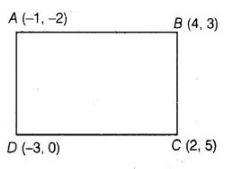# The points A(- 1, – 2), B (4, 3),

Question:

The points A(- 1, – 2), B (4, 3), C (2, 5) and D (- 3, 0) in that order form a rectangle.

Solution:

TrueDistance between $A(-1,-2)$ and $B(4,3)$,

$A B=\sqrt{(4+1)^{2}+(3+2)^{2}}$

$=\sqrt{5^{2}+5^{2}}=\sqrt{25+25}=5 \sqrt{2}$

Distance between $C(2,5)$ and $D(-3,0)$,

$C D=\sqrt{(-3-2)^{2}+(0-5)^{2}}$

$=\sqrt{(-5)^{2}+(-5)^{2}}$

$=\sqrt{25+25}=5 \sqrt{2}$

$\left[\because\right.$ distance between the points $\left(x_{1}, y_{1}\right)$ and $\left.\left(x_{2}, y_{2}\right), d=\sqrt{\left(x_{2}-x_{1}\right)^{2}+\left(y_{2}-y_{1}\right)^{2}}\right]$

Distance between $A(-1,-2)$ and $D(-3,0)$.

$A D=\sqrt{(-3+1)^{2}+(0+2)^{2}}=\sqrt{(-2)^{2}+2^{2}}=\sqrt{4+4}=2 \sqrt{2}$

and distance between $B(4,3)$ and $C(2,5), B C=\sqrt{(4-2)^{2}+(3-5)^{2}}$

$=\sqrt{2^{2}+(-2)^{2}}=\sqrt{4+4}=2 \sqrt{2}$

We know that, in a rectangle, opposite sides and equal diagonals are equal and bisect each other.

Since $A B=C D$ and $A D=B C$

Also, distance between $A(-1,-2)$ and $C(2,5), A C=\sqrt{(2+1)^{2}+(5+2)^{2}}$

$=\sqrt{3^{2}+7^{2}}=\sqrt{9+49}=\sqrt{58}$

and distance between $D(-3,0)$ and $B(4,3), D B=\sqrt{(4+3)^{2}+(3-0)^{2}}$

$=\sqrt{7^{2}+3^{2}}=\sqrt{49+9}=\sqrt{58}$

Since, diagonals AC and BD are equal.

Hence, the points A (-1, – 2), B (4, 3), C (2, 5)and D (- 3 0)form a rectangle.